Get inspired by the success stories of our students in IIT JAM MS, ISI  MStat, CMI MSc DS.  Learn More

# ISI MStat PSB 2009 Problem 6 | abNormal MLE of NormalThis is a very beautiful sample problem from ISI MStat PSB 2009 Problem 6. It is based on the idea of Restricted Maximum Likelihood Estimators, and Mean Squared Errors. Give it a Try it !

## Problem-ISI MStat PSB 2009 Problem 6

Suppose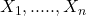are i.i.d.,, where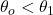are two specified numbers. Find the MLE of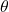and show that it is better than the sample mean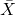in the sense of having smaller mean squared error.

### Prerequisites

Maximum Likelihood Estimators

Normal Distribution

Mean Squared Error

## Solution :

This is a very interesting Problem ! We all know, that if the condition ", for some specified numbers" had been not given, then the MLE would have been simply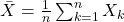, the sample mean of the given sample. But due to the restriction overthings get interestingly complicated.

So, simplify a bit, lets write the Likelihood Function of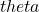given this sample,,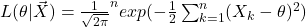, whenow taking natural log both sides and differentiating, we find that ,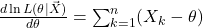.

Now, verify that if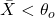, then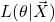is always a decreasing function of, [ where, $$\theta_o \le \theta \le \theta_1$$], Hence the maximum likelihood attains at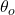itself. Similarly, when,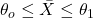, the maximum likelihood attains at, lastly the likelihood function will be increasing, hence the maximum likelihood will be found at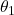.

Hence, the Restricted Maximum Likelihood Estimator of, sayNow, to check that,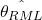is a better estimator than, in terms of Mean Squared Error (MSE).

Now,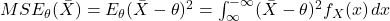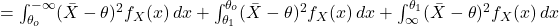.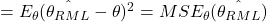.

Hence proved !!

## Food For Thought

Now, can you find an unbiased estimator, for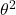?? Okay!! now its quite easy right !! But is the estimator you are thinking about is the best unbiased estimator !! Calculate the variance and also compare weather the Variance is attaining Cramer-Rao Lowe Bound.

Give it a try !! You may need the help of Stein's Identity.

## Subscribe to Cheenta at Youtube

This is a very beautiful sample problem from ISI MStat PSB 2009 Problem 6. It is based on the idea of Restricted Maximum Likelihood Estimators, and Mean Squared Errors. Give it a Try it !

## Problem-ISI MStat PSB 2009 Problem 6

Supposeare i.i.d.,, whereare two specified numbers. Find the MLE ofand show that it is better than the sample meanin the sense of having smaller mean squared error.

### Prerequisites

Maximum Likelihood Estimators

Normal Distribution

Mean Squared Error

## Solution :

This is a very interesting Problem ! We all know, that if the condition ", for some specified numbers" had been not given, then the MLE would have been simply, the sample mean of the given sample. But due to the restriction overthings get interestingly complicated.

So, simplify a bit, lets write the Likelihood Function ofgiven this sample,,, whenow taking natural log both sides and differentiating, we find that ,.

Now, verify that if, thenis always a decreasing function of, [ where, $$\theta_o \le \theta \le \theta_1$$], Hence the maximum likelihood attains atitself. Similarly, when,, the maximum likelihood attains at, lastly the likelihood function will be increasing, hence the maximum likelihood will be found at.

Hence, the Restricted Maximum Likelihood Estimator of, sayNow, to check that,is a better estimator than, in terms of Mean Squared Error (MSE).

Now,..

Hence proved !!

## Food For Thought

Now, can you find an unbiased estimator, for?? Okay!! now its quite easy right !! But is the estimator you are thinking about is the best unbiased estimator !! Calculate the variance and also compare weather the Variance is attaining Cramer-Rao Lowe Bound.

Give it a try !! You may need the help of Stein's Identity.

## Subscribe to Cheenta at Youtube

This site uses Akismet to reduce spam. Learn how your comment data is processed.

### Knowledge Partner